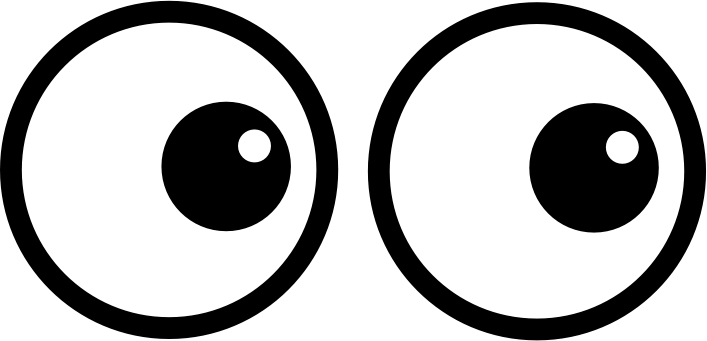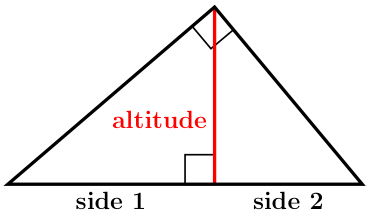# GEOM 2 | Lesson 4 | Watch (Triangle Properties)# Triangle Properties

Here are visuals for each theorem:

##### Angle Bisector Theorem:
• $$\dfrac{\overline{CA}}{\overline{CD}}=\dfrac{\overline{BA}}{\overline{DB}}$$##### Median Theorem of a Triangle:

Concurrency and medians theorem

The distance from a vertex to the centroid is two-thirds the length of the median.If $$\overline{AF}$$, $$\overline{BE}$$ and $$\overline{CD}$$ are medians, then $$AG=\dfrac{2}{3} AF$$, $$BG=\dfrac{2}{3}BE$$ and $$CG=\dfrac{2}{3} CD$$.

##### Perpendicular Bisector Theorem:

Theorem: Concurrency of Perpendicular Bisectors of a Triangle

• The perpendicular bisectors of a triangle intersect at a point that is equidistant from the vertices of a triangle.
• $$PA=PB=PC$$##### Altitude of a Triangle:

An altitude of a triangle is a perpendicular segment that joins a vertex of the triangle to the opposite side.• $$\dfrac{\text{side 1}}{\color{red}{altitude}}=\dfrac{\color{red}{altitude}}{\text{side 2}}$$